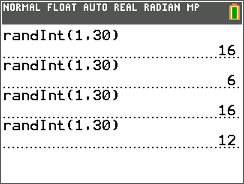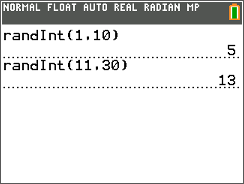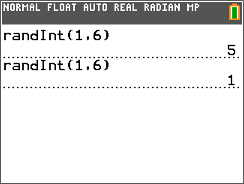# Activities

••• ##### Subject Area

• Math: Statistics: Sampling Distributions

• ##### Author9-12

25 Minutes

• ##### Device
• TI-84 Plus
• TI-84 Plus Silver Edition
•TI-84 Plus C Silver Edition
•TI-84 Plus CE
• ##### Report an Issue

Sampling#### Activity Overview

Students learn about each of the four types of random sampling methods and use the randInt command to find each kind of sample from a given population.

#### Key Steps

•In this activity, students will explore probability sampling methods. They discuss populations, samples, and what it means for a sample to be representative of the population.

Students will use the random integer command to investigate four types of probability samples. They use the numbers 1 to 30 to represent 30 people and then generate 8 random numbers for a Simple Random Sample.

•Students discuss a stratified random sample, often just called a stratified sample. They find a random sample of 12 people from a list, where the list has been divided into two unequal groups. They use randint(1,10) until they have six women and then randint(11, 30) until they have six men. They will discuss why this is not a representative sample and how they can change it to make it representative.

•Students will then create a cluster sample and a systematic sample, where the cluster or the starting point is selected using the random integer command. At the end of the activity, they will be able to discuss the advantages and disadvantages of each sampling method.